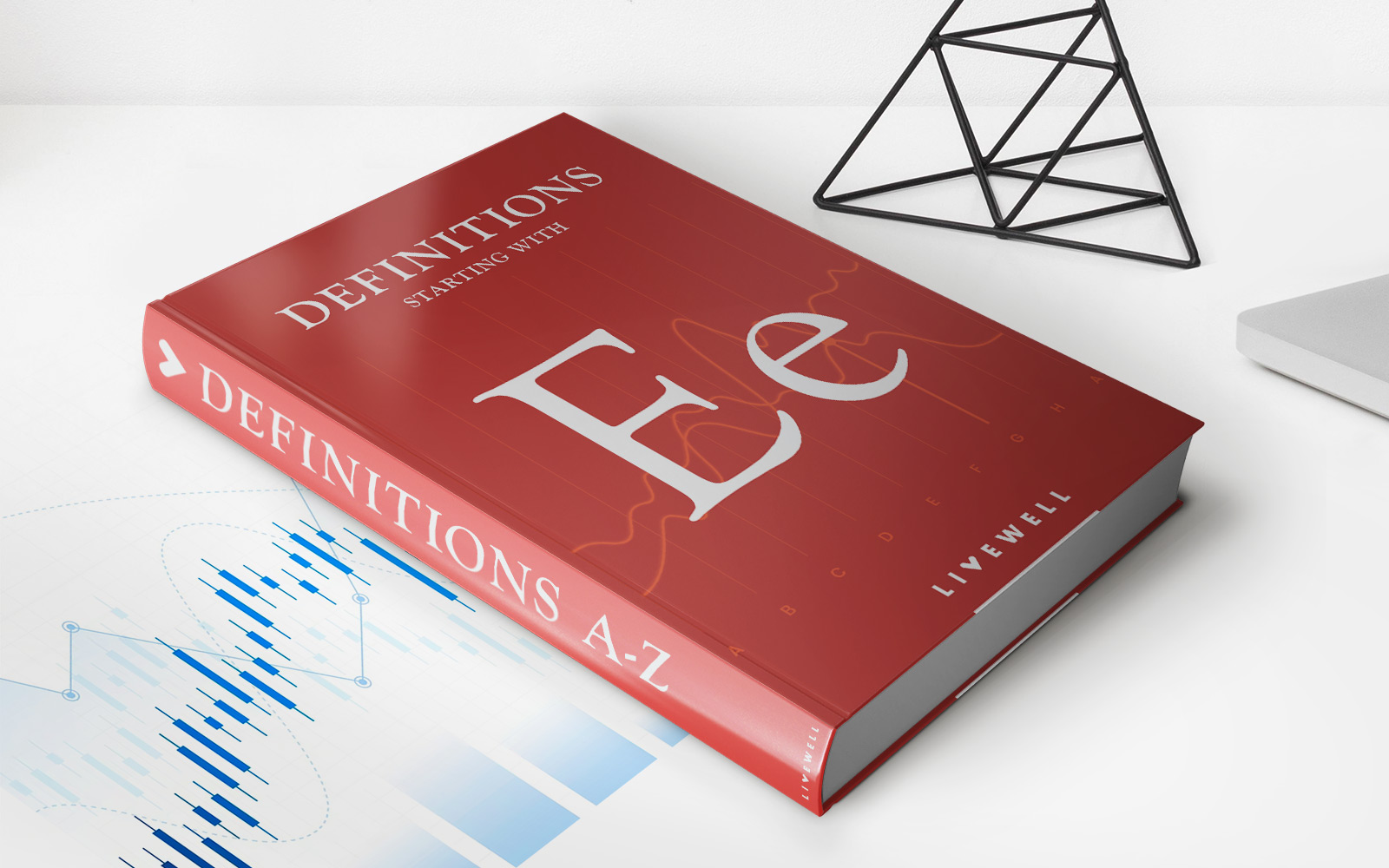Home>Finance>Effective Annual Interest Rate: Definition, Formula, And ExampleFinance

# Effective Annual Interest Rate: Definition, Formula, And Example

Learn about the definition, formula, and example of effective annual interest rate in finance. Understand how it impacts your investments and borrowing decisions.

## The Effective Annual Interest Rate: A Complete Guide

Welcome to our finance category! Today, we’re going to dive into the world of interest rates and explore one important concept: the Effective Annual Interest Rate (EAR). If you’ve ever wondered how interest rates are calculated or wanted to compare different financial products, understanding the EAR is crucial. In this blog post, we’ll define the EAR, explain the formula for calculating it, and provide a practical example to help you grasp its significance.

## Key Takeaways:

• The Effective Annual Interest Rate (EAR) takes compounding into account, giving you a more accurate measure of the true cost or return on a loan or investment.
• The EAR is calculated by considering the stated annual interest rate and the compounding periods within a year.

## 1. What is the Effective Annual Interest Rate?

The Effective Annual Interest Rate, often referred to as the Annual Equivalent Rate (AER) or Annual Percentage Yield (APY), is a way to express the actual interest rate over a period of one year. It accounts for both the stated interest rate and the frequency of compounding.

Let’s break this down further:

• The stated annual interest rate is the advertised interest rate, typically expressed as a percentage, that a lender or institution offers on a loan or the return on an investment.
• Compounding is the process of accumulating interest on the initial principal amount and any previously earned interest. It can be done annually, semi-annually, quarterly, monthly, or even daily, depending on the terms of the financial product.

Now, you might be wondering why the Effective Annual Interest Rate is important and why it differs from the stated rate. The answer lies in the power of compounding.

## 2. How to Calculate the Effective Annual Interest Rate

To calculate the Effective Annual Interest Rate, you can use the following formula:

EAR = (1 + r/n)^n – 1

Where:

• r represents the stated annual interest rate in decimal form (divide the advertised rate by 100).
• n denotes the number of compounding periods within one year.

Let’s put this formula into practice with an example:

Suppose you deposit \$1,000 in a savings account with an annual interest rate of 5%, compounded quarterly (four times a year). By using the formula, we can calculate the Effective Annual Interest Rate:

EAR = (1 + 0.05/4)^4 – 1 = 0.050933

So, the effective annual interest rate for this savings account is approximately 5.0933%.

## Wrapping Up

The Effective Annual Interest Rate is a powerful tool that allows you to gain a better understanding of the true cost or return on a loan or investment. By considering the stated rate and the frequency of compounding, you can compare financial products more accurately and make informed decisions about your personal or business finance.

Remember, compounding can significantly impact your financial outcomes, so it’s always essential to evaluate the Effective Annual Interest Rate when assessing different financial options.

We hope this blog post has shed some light on the concept of the Effective Annual Interest Rate. Stay tuned for more finance-related content as we unravel the mysteries of the financial world. Happy exploring!

• https://livewell.com/finance/effective-annual-interest-rate-definition-formula-and-example/